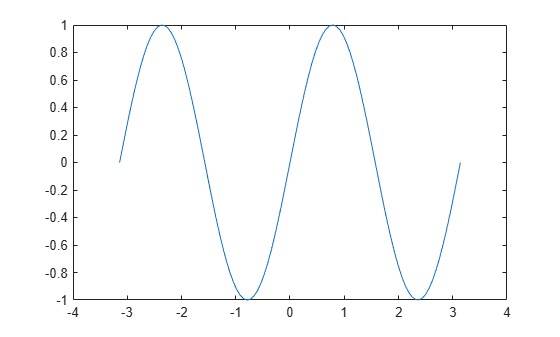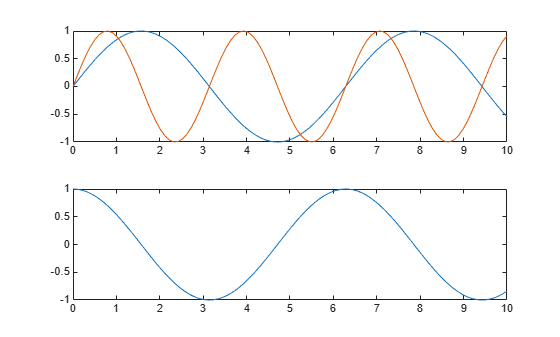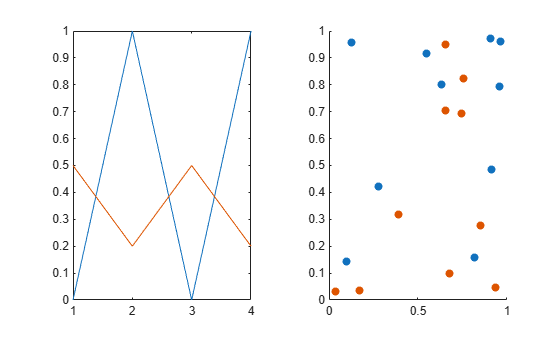hold

Retain current plot when adding new plots

Description

example

hold on retains plots in the current axes so that new plots added to the axes do not delete existing plots. New plots use the next colors and line styles based on the ColorOrder and LineStyleOrder properties of the axes. MATLAB® adjusts axes limits, tick marks, and tick labels to display the full range of data. If axes do not exist, then the hold command creates them.

example

hold off sets the hold state to off so that new plots added to the axes clear existing plots and reset all axes properties. The next plot added to the axes uses the first color and line style based on the ColorOrder and LineStyleOrder properties of the axes. This option is the default behavior.

hold all is the same as hold on. This syntax will be removed in a future release. Use hold on instead.

hold toggles the hold state between on and off.

example

hold(ax,___) sets the hold state for the axes specified by ax instead of the current axes. Specify ax before all other arguments in any of the previous syntaxes. Use single quotes around the 'on' and 'off' inputs, such as hold(ax,'on').

Examples

collapse all

Create a line plot. Use hold on to add a second line plot without deleting the existing line plot. The new plot uses the next color and line style based on the ColorOrder and LineStyleOrder properties of the axes. Then reset the hold state to off.

x = linspace(-pi,pi);
y1 = sin(x);
plot(x,y1)

hold on
y2 = cos(x);
plot(x,y2)
hold offWhen the hold state is off, new plots delete existing plots. New plots start from the beginning of the color order and line style order.

y3 = sin(2*x);
plot(x,y3)Starting in R2019b, you can display a tiling of plots using the tiledlayout and nexttile functions. Call the tiledlayout function to create a 2-by-1 tiled chart layout. Call the nexttile function to create the axes objects ax1 and ax2. Plot a sine wave plot in each axes.

x = linspace(0,10);
y1 = sin(x);
y2 = cos(x);
tiledlayout(2,1)

% Top plot
ax1 = nexttile;
plot(ax1,x,y1)

% Bottom plot
ax2 = nexttile;
plot(ax2,x,y2)Add a second sine wave to the top axes.

hold(ax1,'on')
y3 = sin(2*x);
plot(ax1,x,y3)
hold(ax1,'off')Create a 1-by-2 tiled chart layout. Call the nexttile function to create two axes objects and plot into the axes.

t = tiledlayout(1,2);
ax1 = nexttile;
ax2 = nexttile;
plot(ax1,[0 1 0 1])
scatter(ax2,rand(1,10),rand(1,10),'filled')Set the hold state for both of the axes to 'on'. Then display additional data in each plot.

hold([ax1 ax2],'on')
plot(ax1,[.5 .2 .5 .2])
scatter(ax2,rand(1,10),rand(1,10),'filled')Input Arguments

collapse all

Target axes, specified as one of the following:

• Any type of axes object: an Axes, PolarAxes, or GeographicAxes object.

• An array of axes objects that belong to the same class. To determine the class, use the class function.

If you do not specify the axes, then hold sets the hold state for the current axes.

Tips

• Use the ishold function to test the hold state.

Algorithms

The hold function sets these properties:

• NextPlot axes property - Sets this property of the associated Axes, PolarAxes, or GeographicAxes object to either 'add' or 'replace'.

• NextPlot figure property - Sets this property of the Figure object to 'add'.